New update is available. Click here to update.

# Label Encoding of Dataset

## Introduction

Machine Learning is a data-driven technology. This data is used to train Machine Learning Models. But data processing and computation happened even before we had this technology. Hence, the data is captured in a way that it’s convenient for humans to interpret. But it might not be as readable and convenient for machines. Humans can process numbers, string values, and other various data types and data formats. Unfortunately, it’s not the same with machines. Hence, this data needs to be preprocessed before it can be employed for training. There are various stages and techniques in the data preprocessing phase. One such technique is Label Encoding which would be the focus of this blog. We have a blog specifically covering the data preprocessing phase in model training. You may want to check that out as well later.

## Categorical Data Encoding - Label Encoding

Let us first understand what is meant by Data Encoding. A data set is essentially a collection of different feature values for a number of entries. These feature values can be either continuous or categorical. The categorical variables can be encoded. Data encoding means mapping all the possible values in a categorical feature to a corresponding numeric value. See the example given below:-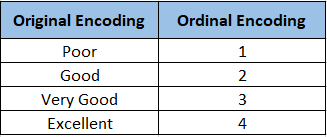As we can see, the original feature had 4 discrete values, namely- Poor, Good, Very Good, and Excellent. So, all the discrete values were mapped to a distinct numeral.

‘Poor’ to 1

‘Good’ to 2

‘Very Good’ to 3

‘Excellent’ to 4

This is an example of Label Encoding. There are other different data encoding techniques like One-hot Encoding, Hash Encoding, etc. We’ll learn how Label encoding is different from other encoding techniques later in the blog.

## Why encode the data?

In the previous example, we saw how Label encoding works. Now the question arises, why do we need to encode these categorical features? We learned how machines can’t process certain data types efficiently during the training phase. String variables are one of those data types. Machines are good at processing numeric data. However, string variables can’t be dealt with directly. Hence, we mapped each of the discrete string values (Poor, Good, Very Good, and Excellent) to a unique numeral(1, 2, 3, 4).

## When to use Label Encoding?

We learned about Label Encoding. But there is another popular data encoding technique, One-hot Encoding. See the Example given below:-

One-hot Encoding creates dummy columns for every discrete value in the categorical feature. If the value is present in the original feature column, the corresponding dummy column is activated as 1. If not, then it stays 0.

This is another way of categorical data encoding. So which one to choose? It depends on the kind of feature column being encoded. If the values show some order within them, like the example above (Poor<Good<Very Good<Excellent), then Label encoding is preferred. If the values don’t have an order among them, one-hot Encoding is preferred. For example, a categorical feature column consisting of 5 different city names - New Delhi, Mumbai, Bangalore, Pune, and Kolkata. These discrete values don’t have any order among them, unlike the previous example.

Label Encoding is preferred in ordered values in a feature because it assigns a unique numeric value to every value in the dataset- Poor - 1, Good - 2, Very Good - 3, Excellent - 4. Now, It may be that during training, the model may assign a higher weight to a higher numeric value. Therefore, this encoding technique makes sense in this example. But what about the example

with feature column having 5 different city names? City names don’t follow any order among them. Hence it doesn’t make any sense to assign a higher numeric value to any of them. In this case, One-hot Encoding works better.

## Implementation

Now that we know what Label Encoding is and how it works let’s jump into its Python Implementation. You can find the dataset here

First, we need to import the required libraries and create the data frame.

``````import pandas as pd
import numpy as np
import matplotlib.pyplot as plt
%matplotlib inline``````

``````bigmart = pd.read_csv('train_bm.csv')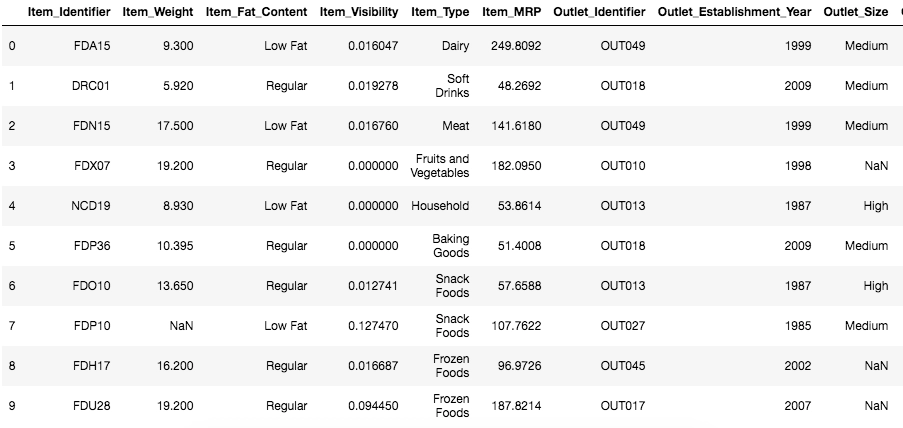For this example, we will encode the feature ‘Outlet_Size’.

As we can see, there are some missing values in the feature. We will fill the missing values with ‘medium’ to proceed further.

``````bigmart['Outlet_Size'].fillna('Medium', inplace=True)
bigmart['Outlet_Size'].unique()``````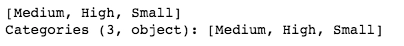There can be 2 approaches to label encode the data -

1. Using category codes approach
``````bigmart['Outlet_Size'] = bigmart['Outlet_Size'].astype('category')
bigmart['Outlet_Size_Cat'] = bigmart['Outlet_Size'].cat.codes
bigmart[['Outlet_Size' , 'Outlet_Size_Cat']]``````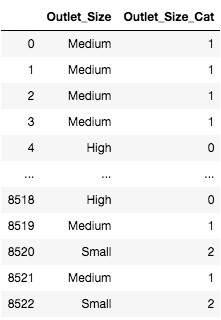2. Using Scikit Learn Library Approach

``from sklearn.preprocessing import LabelEncoder``

``````labelencoder = LabelEncoder()
# Assigning numerical values and storing in another column
bigmart['Outlet_Size_Cat_Sk'] = labelencoder.fit_transform(bigmart['Outlet_Size'])
bigmart[['Outlet_Size','Outlet_Size_Cat','Outlet_Size_Cat_Sk']]``````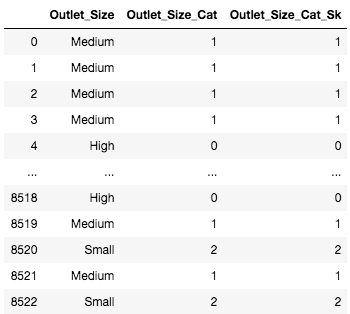You may use either of the methods. As we can see, It doesn’t make any difference in the final outcome.

1. Briefly explain Data encoding and its purpose.
Ans. Data Encoding is a technique in which categorical variables in a feature are mapped to distinct numerical values. This is a necessary step in the data preprocessing stage since the string values cannot be processed efficiently by the training model. Two of the most popular encoding techniques are Label Encoding and One-hot Encoding.

2. Given a feature with 4 categorical variables namely - Slow, Average, Fast, Very Fast, which encoding technique are you most likely to use?
Ans. Label Encoding since the variables have a hierarchical order between them.

3. Mention some of the Data encoding techniques.
Ans. Label Encoding, One-hot Encoding, Hash Encoding, Dummy Encoding, etc.

## Key Takeaways

Label Encoding is very commonly used in any machine learning model. Hence, it’s essential to have a good command of this technique. This blog thoroughly covers Label Encoding- What is it, its purpose, and when it’s employed. We suggest implementing the code yourself for a better understanding of the topic. You can check out our industry-oriented data science course, curated by Stanford alumnus and industry experts.

Happy Learning!!